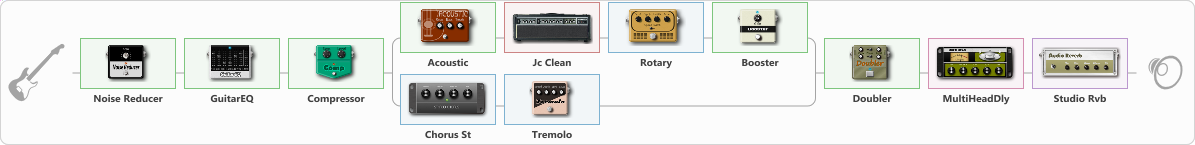# Floods (clean tone)

Discussion in 'ToneLib-GFX presets' started by daniel54673, Mar 23, 2021.

Tags:
1. Floods (clean tone)

Preset name: Floods Clean

Effects chain:Effect: "Noise Reducer" (Dynamics / Filter), active - "yes"
{
"Sens" = 50
"Mode" = Hard
}

Effect: "GuitarEQ" (Dynamics / Filter), active - "yes"
{
"160 Hz" = -5
"400 Hz" = -4
"800 Hz" = -4
"1.6 kHz" = 4
"3.2 kHz" = 5
"6.4 kHz" = 10
"12 kHz" = -2
"Level (dB)" = 0
}

Effect: "Compressor" (Dynamics / Filter), active - "yes"
{
"Sense" = 31
"Level" = 50
}

Effect: "Splitter" (Dynamics / Filter), active - "yes"
{
"A-Bypass" = Off
"A-Pan" = 0
"A-Level" = 55
"B-Bypass" = Off
"B-Pan" = 30
"B-Level" = 60

'A' branch:
{

Effect: "Acoustic" (Dynamics / Filter), active - "yes"
{
"Style" = Arpeggio/Classic
"Resonance" = 100
"Bass" = 37
"Treble" = 45
}

Effect: "Jc Clean" (Amp simulators), active - "yes"
{
"Gain" = 50
"Bass" = 30
"Middle" = 70
"Treble" = 40
"Presence" = 50
"Master" = 50
"Level (dB)" = 0
}

Effect: "Rotary" (Modulation / Sfx), active - "yes"
{
"Speed A" = 0.8
"Speed B" = 0.8
"SpeedSw" = B
"Depth" = 38
"Accel" = 3.7
}

Effect: "Booster" (Dynamics / Filter), active - "yes"
{
"Gain" = 60
}
}
'B' branch:
{

Effect: "Chorus St" (Modulation / Sfx), active - "yes"
{
"Speed" = 1.9
"Depth" = 31
"Center" = 4.4
"Mix" = 40
}

Effect: "Tremolo" (Modulation / Sfx), active - "yes"
{
"Speed" = 9.2
"Depth" = 42
"Level" = 50
}
}
}

Effect: "Doubler" (Dynamics / Filter), active - "yes"
{
"A-Dly" = 75
"A-Inv" = On
"A-Level" = 75
"B-Dly" = 50
"B-Inv" = Off
"B-Level" = 50
}

Effect: "MultiHeadDly" (Delay), active - "yes"
{
"Time" = Note sync,
"Feedback" = 50
"Tone" = 60
"MODE" = Conventional echo
"Mix" = 60
}

Effect: "Studio Rvb" (Reverberation), active - "yes"
{
"Time" = 7.4
"PreDelay" = 32
"LoDamp" = 0
"HiDamp" = 12
"Mix" = 58
}

Note: You will need to download and install the ToneLib-GFX software to use the preset.

#### Attached Files:

• ###### Floods_clean_tone.tlgfx
File size:
1.1 KB
Views:
3,370
stek, Jack Disney, Onur and 13 others like this.